# Calculating Electric Power

## Chapter 2 - Ohm's Law

### Learn the Power Formula

We’ve seen the formula for determining the power in an electric circuit: by multiplying the voltage in “volts” by the current in “amps” we arrive at an answer in “watts.” Let’s apply this to a circuit example: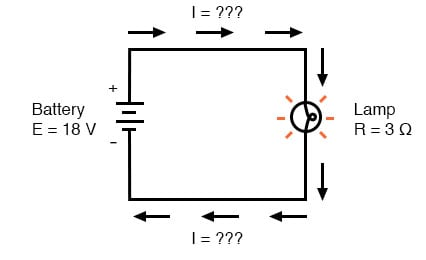### How to Use Ohm’s Law to Determine Current

In the above circuit, we know we have a battery voltage of 18 volts and a lamp resistance of 3 Ω. Using Ohm’s Law to determine current, we get: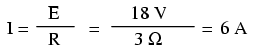Now that we know the current, we can take that value and multiply it by the voltage to determine power: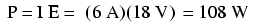This tells us that the lamp is dissipating (releasing) 108 watts of power, most likely in the form of both light and heat.

### Increasing the Battery’s Voltage

Let’s try taking that same circuit and increasing the battery’s voltage to see what happens. Intuition should tell us that the circuit current will increase as the voltage increases and the lamp resistance stays the same. Likewise, the power will increase as well: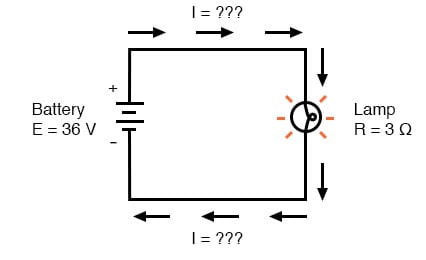Now, the battery’s voltage is 36 volts instead of 18 volts. The lamp is still providing 3 Ω of electrical resistance to the flow of current. The current is now: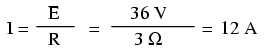This stands to reason: if I = E/R, and we double E while R stays the same, the current should double. Indeed, it has: we now have 12 amps of current instead of 6. Now, what about power?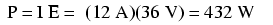### What does Increasing a Battery’s Voltage do to Power?

Notice that the power has increased just as we might have suspected, but it increased quite a bit more than the current. Why is this? Because power is a function of voltage multiplied by current, and both voltage and current doubled from their previous values, the power will increase by a factor of 2 x 2, or 4. You can check this by dividing 432 watts by 108 watts and seeing that the ratio between them is indeed 4. Using algebra again to manipulate the formula, we can take our original power formula and modify it for applications where we don’t know both voltage and current: If we only know voltage (E) and resistance (R):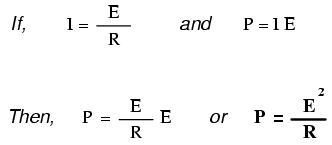If we only know current (I) and resistance (R):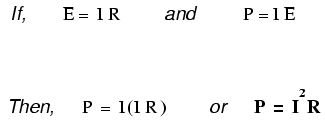### Joule’s Law Vs. Ohm’s Law

A historical note: it was James Prescott Joule, not Georg Simon Ohm, who first discovered the mathematical relationship between power dissipation and current through a resistance. This discovery, published in 1841, followed the form of the last equation (P = I2R), and is properly known as Joule’s Law. However, these power equations are so commonly associated with the Ohm’s Law equations relating voltage, current, and resistance (E=IR ; I=E/R ; and R=E/I) that they are frequently credited to Ohm.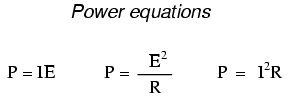REVIEW:

• Power measured in watts, symbolized by the letter “W”.
• Joule’s Law: P = I2R ; P = IE ; P = E2/R

RELATED WORKSHEETS:

Try out our Ohm’s Law Calculator in our Tools section.

• Share
Published under the terms and conditions of the Design Science License